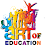-->

# STD 3 MATHS UNIT 5 આકારો અને ભાત ઓનલાઈન ટેસ્ટ NCERT Solutions For Class 3 Maths Chapter 5 Shapes and Designs

### Maths NCERT Solutions for Class 3 Maths Chapter 5 Shapes and Designs

HAVE FUN WITH SHAPES

Question 1:

Colour the clown following the directions given below: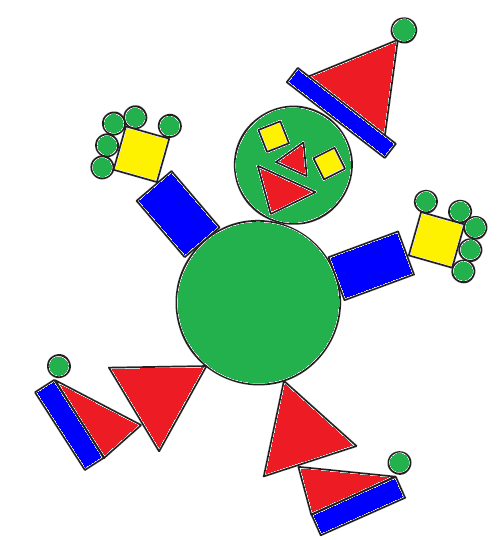Question 2:

How many triangles are there in the following figures?

(i) 12 triangles

(ii) 11 triangles

(iii) 13 triangles

Question 3:

Find the biggest rectangle in the figures given below.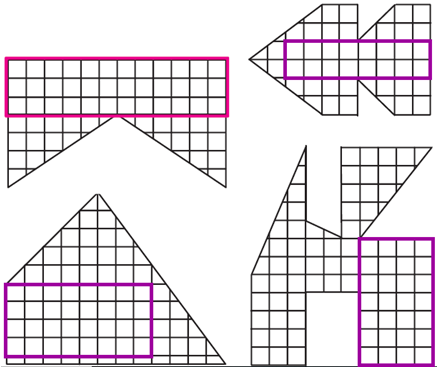EDGES AND CORNERS

Question 1:

a) Looking at the picture given above, can you tell who is out?

b) Where is Guddu standing?

c) Can this game be played around a round table? Why?

a) Guddu is out.

b) Guddu is standing in the middle of one edge of the table.

c) No, this game cannot be played around a round table because the round table will not have any corner.

Question 2:

a) Look around you and identify things with straight and curved edges.

b) Do the things with straight edges have corners?

c) Do the things with curved edges have corners?

d) Try to find things which have both straight and curved edges.

a) Things with straight edges: computer screen, table, book, notepad, wall.

Things with curved edges: football, lemon, coconut,

b) Yes, things with the straight edges have corners.

c) No, things with curved edges have corners do not have corners.

d) Things having both straight and curved edges: screw driver, electric guitar, bicycle, car, aeroplane.

ACTIVITY TIME

Question 1 – 5:

1. Take a rectangular sheet of paper.

2. Count its corners.

3. Now fold one of its corners.

a) How many corners does it have now?

b) How many corners will you get by folding?

i) 2 corners

ii) 3 corners

iii) 4 corners

c) Can you fold this paper in such a way that it has only three corners? You are allowed only two folds. What shape will you get?

4. Repeat the activity with a square sheet of paper.

5. Can you fold all the corners of the square sheet in such a way that the number of corners remains unchanged?

1. Kids have to do as directed.

2. There are 4 corners in the paper.

3. (a) Now, there are 5 corners in the paper.

(b) (i) 6 corners

(ii) 7 corners

(iii) 8 corners

(c) Yes, the paper can be folded in such a way that it has only three corners, using 2 folds. We will get a triangle.

4. Kids have to do as directed.

5. Yes, the corners of the square can be folded in such a way that the number of corners remains unchanged, i.e. 4.

Question:

Look at the following table and tick the names of things that have corners. Also count the number of edges and corners in each of them.

 Name of thing Whether it has corners Number of edges Number of corners Die Yes 12 8 Ball No 0 0 Eraser Yes 12 8 Egg No 0 0 Sheet of paper Yes 4 4

Question:

In the following figures, tick ( ) those which have corners. Do these figures have curved lines?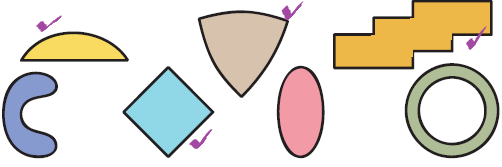Yes, these figures have curved line also.

Question:

Using only straight lines, can you draw a figure which has no corners?

No, we can’t draw a figure which has no corners.

TANGRAM

Question 1:

How many triangles do you have in your set? Are all of them equal in size? Find out.

There are 3 triangles in the given set, the triangles are named 1, 2 and 5. No all of them are not equal in size.

Question 2:

Use the two small triangles in the tangram set to get the following shapes: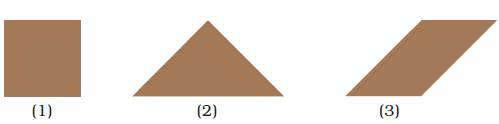Do as directed, using the triangles numbered 2 and 5.

Question 3:

Which two pieces of the tangram set are exactly same? Find out.

The triangles 2 and 5 are exactly same.

Question 4:

Take pieces 4 and 5 from the set and find out on which side of the triangle you can join the other piece.

We can join the piece numbered 4 on the slant side of the piece numbered 5.

Question 5:

Find matching sides among the following pairs of pieces.

a) Pieces 1 and 2

b) Pieces 2 and 4

c) Pieces 1 and 5

d) Pieces 2 and 5

a) The longest side of 2 match with one of the short sides of 1.

b) The longest side of 2 match with the longest side of 4.

c) The longest side of 5 match with one of the short sides of 1.

d) The longest side of 2 match with the longest side of 5, the short side of 2 match with the short side of 5.

WEAVING PATTERNS

Question 1 – 4:

1. Which geometrical shapes can you identify in these borders? Draw them in your notebook.
2. Is any shape repeating in a particular pattern? Which ones?
3. Are the shapes made of (i) Curved lines (ii) Straight lines (iii) Both curved and straight lines
4. Look at your clothes, your mother’s saris/shawls, rugs and mats. Can you identify some patterns? Draw them in your notebook.

1. Geometrical shapes in the pattern: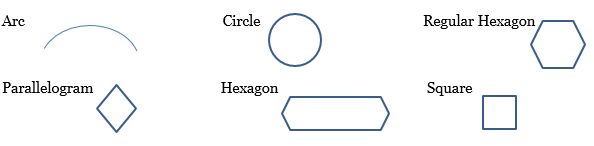1.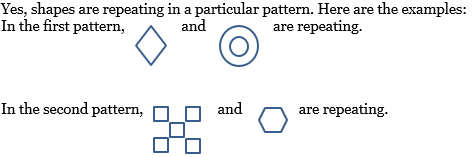2. The shapes are made of both curved and straight lines.
3. Do as directed.

FLOOR PATTERNS

Question 1:

Among the following, can you match the tiles with the designs that they will make on the floor? Draw lines to match.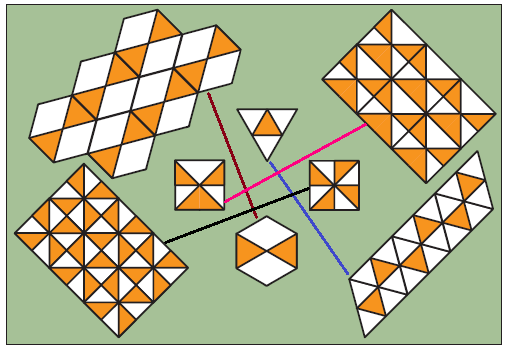Question 2:

Complete the following tiling pattern.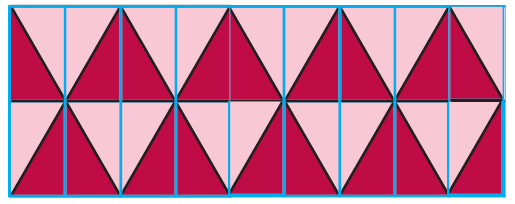Question 3:

Compare it with the pattern on page 70 which also uses six sided shapes. What is the difference between the two?

The pattern on page 70 has hexagons connected to each other. But in the pattern on this page, the hexagons are connected through triangles.

Question 4:

Khushboo and Hariz live in Agra. One day they went to see the Taj Mahal. The floor had the pattern shown below. What do you think? Discuss with your friends.

In the pattern, only one type of tile has been used but at different angles.

### Related Posts

1.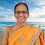Nice online Test
Good Work Sir..

2.Good work sir

1.